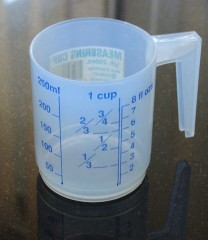# How Many Cups Are In A Gallon?The formula which we commonly use in the English system of measurement to arrive at the relationship between a cup and a gallon are as follows: Three teaspoons (which is abbreviated as three tsp) is equivalent to one tablespoon (which is abbreviated as one tbsp), 16 tablespoons (which is abbreviated as 16 tbsp) is equivalent to one cup (which is abbreviated as one c).

Eight ounces (which is abbreviated as eight oz) is also equivalent to one cup (which is abbreviated as one c), two cups (which is abbreviated as two c) is equivalent to one pint (which is abbreviated as one pt), two pints (which is abbreviated as two pts) is equivalent to one quart (which is abbreviated as one qt) and four quarts (which is abbreviated as four qts) is equivalent to one gallon (which is abbreviated as one gal).

Therefore the calculation which we use to convert cups to gallons is taken as x (the unknown cups, which is denoted in algebra by the variable x) divided by four quarts (which is one gallon) is equivalent to four cups divided by one quart. If the denominator, which is one quart, is multiplied by four, it gives four quarts, which is equivalent to one gallon.

By the same algebraic solution, if the numerator, which is four cups, is multiplied by four, it gives 16 cups, which is equivalent to one gallon. Therefore one gallon is roughly equivalent to sixteen cups.
thanked the writer.
There are 16 cups to a gallon.
thanked the writer.
3 1/4 cups equals 3/4 of a cup.
thanked the writer.
16 cups in a gallon
thanked the writer.
Theirs 16 cups to make a gallon
thanked the writer.
There are 16 cups in a gallon.
thanked the writer.
16
thanked the writer.
16
thanked the writer.
16
thanked the writer.
16
thanked the writer.
8 ounce cups make a gallon of milk
thanked the writer.